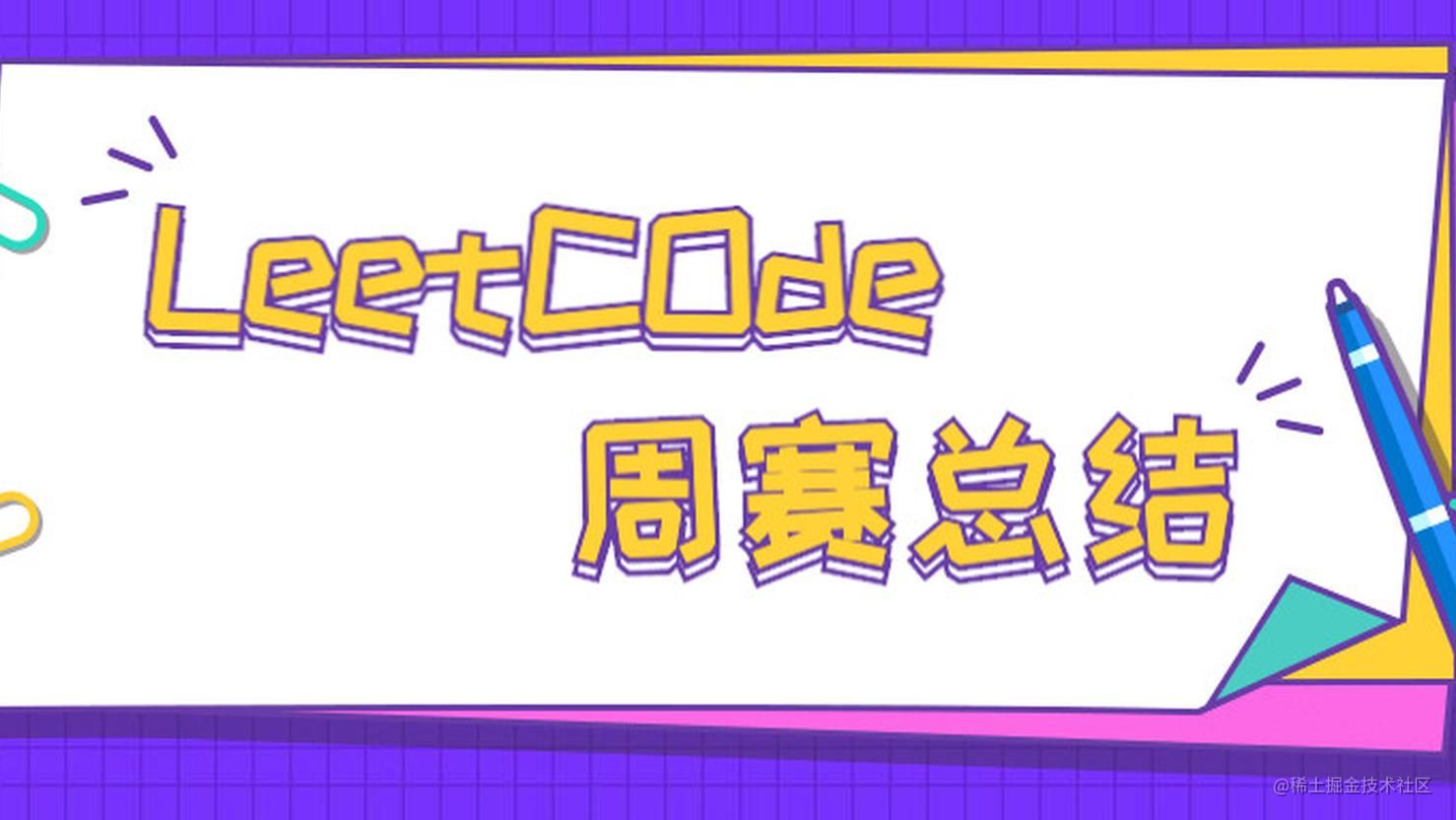前端工程师的 LeetCode 之旅 -- 周赛 20101 整理字符串

• 数据结构 -- 栈

• 利用 charCode 的差值来判断相邻两字符是否互为大小写字符

const makeGood = function(s{
const stack = [];
let startIndex = 0;
while (startIndex < s.length) {
const currentItem = s[startIndex];
if (stack.length && Math.abs(currentItem.charCodeAt(0) - stack[stack.length - 1].charCodeAt(0)) === 32) {
stack.pop();
} else {
stack.push(currentItem);
}
startIndex++;
}

return stack.join('');
};

02 找出第N个字符串中的第K位

• 每一行的字符串的长度为 2^n - 1

• 后一行与前一行是存在递推关系

• 每一行的左右两部分存在翻转关系

const findKthBit = function(n, k{
let current = n;
let reverseCount = 0;
if (n === 1) {
return '0'
}
while (current > 1) {
const total = 2 ** current - 1;
const mid = Math.ceil(total / 2);
if (k === mid) {
if (reverseCount % 2 === 0) {
return '1';
}
return '0';
}
if (k > mid) {
reverseCount++;
k = mid * 2 - k;
}
current--;
}
if (reverseCount % 2 === 0) {
return '0';
}
return '1';
};

03 最大数目不重叠非空子数组

• 子数组目标和值

• 子数组不重叠

const maxNonOverlapping = function(nums, target{
let record = new Set();
let ans = 0;
let currentSum = 0;
for (let i = 0; i < nums.length; i++) {
currentSum += nums[i];
if (record.has(currentSum - target)) {
ans++;
record.clear();
currentSum = 0;
} else {
}
}
return ans;
};

04 切棍子的最小成本

• 当前棍子的长度

• 分割后左半部分棍子后续切割的成本

• 分割后右半部分棍子后续切割的成本

const minCost = function(n, cuts{
const cache = new Map();
return help(0, n, cuts, cache);
};

function help(left, right, cuts, cache{
const cacheKey = `\${left}-\${right}`;
if (cache.has(cacheKey)) {
return cache.get(cacheKey);
}
let min = Number.MAX_SAFE_INTEGER;
for (let i = 0; i < cuts.length; i++) {
if (cuts[i] > left && cuts[i] < right) {
let cost = help(left, cuts[i], cuts, cache) + help(cuts[i], right, cuts, cache);
min = Math.min(min, cost);
}
}
if (min === Number.MAX_SAFE_INTEGER) {
min = 0
} else {
min += right - left;
}
cache.set(cacheKey, min);
return min;
}

• cuts 数组是无序的，导致在遍历的过程中很难判断当前的切割点是否在合法的区间内。

• cuts 不包含首尾下标，导致在递归过程中没有起止边界。

const minCost = function(n, cuts{
const record = new Map();
cuts.sort((a, b) => a - b);
cuts.unshift(0);
cuts.push(n);
const maxIndex = cuts.length - 1;
return help(0, maxIndex, cuts, record);
};

function help(leftIndex, rightIndex, cuts, record{
const cacheKey = `\${leftIndex}-\${rightIndex}`
if (record.has(cacheKey)) {
return record.get(cacheKey);
}
const left = cuts[leftIndex];
const right = cuts[rightIndex];

let min = Number.MAX_SAFE_INTEGER;
for (let i = leftIndex + 1; i < rightIndex; i++) {
const cost = help(leftIndex, i, cuts, record) + help(i, rightIndex, cuts, record);
min = Math.min(cost, min);
}

if (min === Number.MAX_SAFE_INTEGER) {
min = 0;
} else {
min += right - left;
}
record.set(cacheKey, min);
return min;
}

05 往期精彩回顾